# Calculate Years & Months of Service in Excel & Google Sheets

This tutorial will demonstrate how to calculate years of service in Excel and Google Sheets.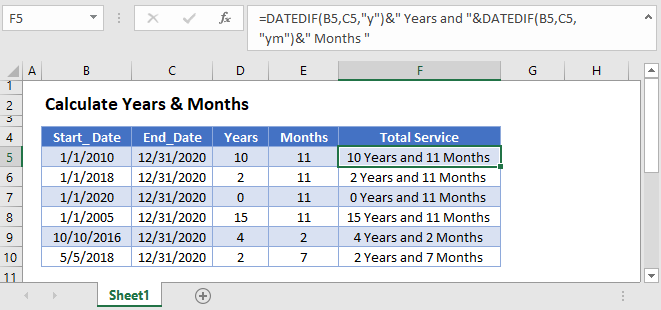## Calculate Years & Months of Service

To calculate years of service, we will use the DATEDIF Function to calculate the number of years and months between dates. Then we will output a readable result by combining the results into a string of text.

### Calculate Years

To calculate the number of years between dates use the DATEDIF Function with criteria “y”:

``=DATEDIF(B3,C3,"y")``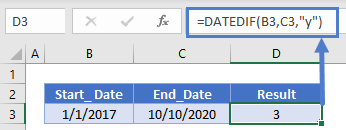### Calculate Months

You can calculate the number of excess months using the criteria “ym” with the DATEDIF Function:

``=DATEDIF(B3,C3,"ym")``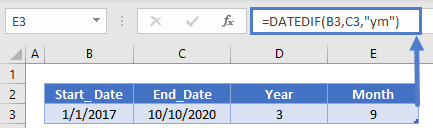### Output Service

Now we can output the years of service in a text string by merging text:

``=DATEDIF(B3,C3,"y")&" Years and "&DATEDIF(B3,C3,"ym")&" Months "``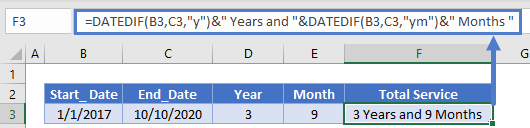### Rounding Service Months

Your service calculation might require rounding months. To round months, you can use the EOMONTH Function to return the last day of a month.

You can round to the previous month:

``=EOMONTH(B3,-1)``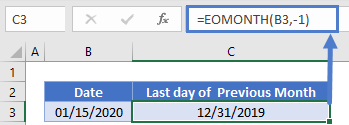Or round to the end of the current month:

``=EOMONTH(B3,0)``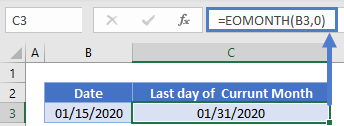By adding 1 to the last day of a month, you can calculate the first day of the next month:

``=EOMONTH(B3,0)+1``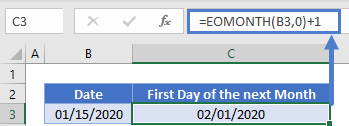With month rounding, you can count partial months as full months, or not count partial months at all.

## Calculate Years & Months of Service in Google Sheets

All of the above examples work exactly the same in Google Sheets as in Excel.### Excel Practice Worksheet

Practice Excel functions and formulas with our 100% free practice worksheets!